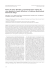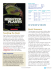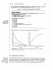# Chaos in a Prey-Predator Model with Infection in

## Transcription

Chaos in a Prey-Predator Model with Infection in
```1
Computational and Mathematical Biology
Chaos in a Prey-Predator Model with Infection in
Predator - A Parameter Domain Analysis
Prasenjit Das, Debasis Mukherjee, Kalyan Das
Abstract
A
prey-predator system with a disease in the predator population is a challenging issue in ecoepidemics which is proposed here and analyzed as a set of three dimensional ordinary nonlinear differential equations. The discrete analogous of the eco-epidemic model is also
investigated. We observed that the system converges to a unique equilibrium point for certain prey
density threshold level and beyond which stability of the system is disturbed. The results of our study
suggest that discrete version of the continuous model exhibits different dynamical complexity
including chaos in real situation.
1 Introduction
Discrete-time population models can exhibit complex
behavior and intriguing dynamics even in the simplest
systems in fluctuating environment. A rigorous study of
mathematical models on biology shows that discretetime models described by difference equations are more
justified than the continuous-time models when the size
of the population is rarely small or populations have
non-overlapping generations. Further, epidemiological
data for infectious diseases is collected in discrete form.
Difference equation models give richer dynamics than
continuous ones for infection disease model. In recent
years diseases in prey-predator systems become most
interesting part of research among all mathematical
models. Such systems governed mainly by continuoustime models and these studies investigated stability,
bifurcation, persistence etc. [3, 4, 7, 8]. However, not
much work has been dealt with the stability analysis of
discrete eco-epidemic models. There are many published
works in mathematical models in ecology and
epidemiology with difference equations for two
equations [1, 2, 5]. The aim of our current study is to
analyze a non-linear system of difference equations with
three set of equations. Most of the eco-epidemic models
consider the disease among the prey species only as
infected prey is more vulnerable to predator except for
those developed by Haque et.al , Venturino  and
Issue 3(4), 2014
Haque . In these three papers, the authors studied the
spread of disease in predator population. Although
parasitic models generally have a tendency to destabilize
the system but study by Hilker et al.  suggests that
predator infection into the system creates paradox of
enrichment whereas its removal develops catastrophic
effect. Here we establish that destabilization which does
not always happen. The disease in predator population
may stabilize the oscillatory prey-predator system.
Another feature of disease transmission in susceptible
predator population allows the prey species to recover.
The effect of disease in our model increases the mortality
rate of predator. The effect of disease in our model
increases the mortality rate of predator which allowing
the stability of the system. The present model focuses
the complex dynamics of discrete version of continuous
eco-epidemic model where predator population is
infected. In Section 2 we present our model.
Boundedness of the system is studied in Section 3 along
with local and global behavior of the continuous-time
model. Asymptotic behavior of discrete version is
carried out in Section 4. In Section 5 numerical examples
and simulations are given and we round up the paper in
Section 6.
2
Computational and Mathematical Biology
2 Mathematical Model
In the prey-predator system where predator is infected
is described by the following equations:
⎡ ⎛
y ⎤
dx
x⎞
= x ⎢r ⎜ 1 − ⎟ −
⎥
dt
k
1
+
x⎦
⎠
⎣ ⎝
⎛ mx
⎞
dy
= y⎜
− β I − c⎟
dt
1
+
x
⎝
⎠
where:
3 Qualitative Analysis of Continuous Model
In this section we study the dynamical behavior of the
continuous-time model.
3.1 Boundedness
It has been shown that, all the solutions of system (1) are
bounded in a positive octant R+3 . The boundedness of
solutions of system (1) is given by the following lemma.
Lemma1. Let µ = min(1,c,d) . Then all the solutions of
for all positive initial values (x0 , y0 , I 0 ) ∈R+3 as t → ∞ .
Issue 3(4), 2014
x̂ =
(1)
where x, y, I denote respectively, the population of prey
species, susceptible predator species and infected
predator species. We assume that the infected predators
do not predate prey which is very much realistic in real
situation. The coefficients r, k, m, β, c and d in model (1)
are all positive constants and their ecological
interpretation are as follows: r represents the intrinsic
growth rate of prey and k denotes the carrying capacity
of prey; m, β, c and d represent the uptake constant of the
predator, effective transmission rate of disease, death
rate of susceptible prey and death rate of infected
predator, respectively. Specific example illustrates the
above situation. Feline Immunodeficiency Virus (FIV)
infects cat population that preys on island bird species.
The reduction of a cat population size due to disease can
help to recover bird species. Another example is
common seal (Phoca Vitulina) is infected by Phocine
Distemper Virus (PDV) which preys on fish. The
infectious agents like the Avian pox, Newcastle disease,
Influenza, Aspergillus’s fumigates etc. affect a wide
range of avian species which preys on insects.
⎧
M(r + 1) ⎫
B = ⎨(x, y, I ) ∈R+3 : 0 ≤ x + y + I ≤
⎬
µ
⎩
⎭
Stability Analysis of Boundary and Positive
Equilibrium
Model system (1) has three boundary equilibriums
namely (i) E0(0, 0, 0) (ii) E1(k, 0, 0) and (iii) E12 ( x̂, ŷ,0)
dI
= I( β y − d)
dt
system (1), will lie in the region:
3.2
c
r{(m − c)k − c}
.
, ŷ =
m−c
km
The local behavior of the model system (1) around each
boundary equilibrium points is stated below:
Proposition1. (i) E0 is unstable, (ii) E1 is locally stable if
mk(1 + k) < c and is unstable otherwise, (iii) E12 is locally
stable if ŷ < min{d / β ,r(1 + x̂)2 / k} . It is easy to see that
system (1) has a unique positive equilibrium E*(x*, y*, I*)
provided either the condition (C1) d/β < r, mx / (1 + x*) >
c or the condition (C2) d/β = r, k > 1 is satisfied, where
r(k − 1) + r 2 (k − 1)2 − 4rk
x =
*
(d − β r)
β
2r
,
d
,
β
1 mx *
I* = (
− c).
β 1 + x*
y* =
We now state the local stability criterion of E*(x*, y*, I*).
Theorem 1: Assume either (C1) or (C2) holds. The
equilibrium point of E*(x*, y*, I*) is locally asymptotically
stable if a11 < 0 and is unstable otherwise, where
x* y *
r
a11 = − x * +
k
(1 + x * )2
We now discuss the global stability of the interior
equilibrium point E*. The global stability condition is
derived using Lyapunov’s direct method. Global
stability is given in the following theorem.
Theorem 2: The interior equilibrium point E*(x*, y*, I*) of
the system (1) is globally asymptotically stable for all
positive initial values (x0 , y0 , I 0 ) ∈R+3 if:
y*
r
.
>
k 1 + x*
3
Computational and Mathematical Biology
Remark: When E* is locally asymptotically stable then it
may not be globally stable, usually in cases with
multiple equilibriums.
p3 = p11p22 p33 + p11p23 p32 + p12 p21p33 .
Further,
p11 = 1 + r −
4 Qualitative Analysis of Discrete Model
In this section we investigate the complex dynamics of
the discrete version of the continuous model (1). The
model is described as follows:
x
,
(1 + x)
my
p21 =
,
(1 + x)2
mx
p22 = 1 +
− β I − c,
(1 + x)
p23 = β y,
p12 =
⎡
⎛
x ⎞
y ⎤
xn+1 = xn ⎢ 1 + r ⎜ 1 − n ⎟ − n ⎥
k
1
+
xn ⎥⎦
⎝
⎠
⎢⎣
⎛
⎞
mxn
yn+1 = yn ⎜ 1 +
− β In − c ⎟
1 + xn
⎝
⎠
(2)
p32 = β I ,
I n+1 = I n (1 + β yn − d)
We first determine the existence of fixed points of
system (2). To determine the fixed points we have to
solve the nonlinear system given by
⎡
⎛
y ⎤
x⎞
x = x ⎢1 + r ⎜ 1 − ⎟ −
⎥
k
1
+
x⎦
⎝
⎠
⎣
⎡
⎤
mx
y = y ⎢1 +
− βI − c⎥
1
+
x
⎣
⎦
I = I[1 + β y − d]
By simple computation of the above algebraic system we
get three non negative fixed points and the positive fixed
point which are as follows: (i) E0(0, 0, 0) (ii) E1(k, 0, 0)
and (iii) E12 ( x̂, ŷ,0) where
c
r{(m − c)k − c}
, ŷ =
m−c
km
x̂ =
provided m > c and (m – c)k > c, (iv) E(x , y , I ) where
x = x ,y = y ,I = I .
*
*
*
We study the asymptotic stability of the system of
difference equations (2) with the help of Schur-Cohn
criterion . That is, asymptotic stability of the system of
difference equations can be found with the eigenvalues
of Jacobean matrix of system (2).
4.1 Stability Analysis of Fixed Point
The characteristic equation of Jacobean matrix of system
(2) at the state variable is:
λ 3 + p1λ 2 + p2 λ + p3 = 0,
p1 = p11 − p22 − p33 ,
p2 = −(p11p22 + p11p33 + p22 p33 + p23 p32 + p12 p21p33 ),
Issue 3(4), 2014
y
2rx
−
,
k (1 + x)2
p33 = 1 + β y − d.
We now state the local behavior of boundary fixed
points.
Proposition2. (i) E0 is unstable, (ii) E1 is locally stable if
mk(1 + k) < c and is unstable if mk(1 + k) > c, (iii) E12 is
locally stable if:
(a)
ŷ
mŷ
r
>
+
,
k (1 + x̂)2 (1 + x̂)3
(b) 4 +
2 x̂ŷ
mx̂ŷ
2rx̂
+
>
, and
k
(1 + x̂)2 (1 + x̂)3
d
,
β
and is unstable otherwise.
Using Schur-Cohn criterion we now state the
following theorem:
(c) ŷ <
Theorem 3: Suppose −1 < a11 < 0 and |p2 − p1 p3 |< 1 − p32
then the fixed point E(x , y , I ) is asymptotically stable.
5 Numerical Examples and Simulations
In this section we provide comparative study with
different numerical scenarios for model systems (1) and
(2) using theoretically picked parameter domain values
for different situations and explaining their complex
dynamical nature. All numerical simulations are
generated by using MATLAB 7.4.0.
4
Computational and Mathematical Biology
Figure 1a
Infected Predator
1.5
1
0.5
0
2
1.5
1.5
1
1
0.5
0.5
0
Susceptible Predator
0
Prey
Figure 1(a): When r = k, for the parameter values r = 3/2, k = 3/2, m = 1, β = 1/4, c = 1/4, d = 1/4, the system (1) has a
unique equilibrium point (1, 1, 1) in the phase portrait which is globally asymptotically stable.
Figure 1b
1.4
Infected Predator
1.2
1
0.8
0.6
0.4
0.2
0
2.5
2
1.5
1.5
1
1
0.5
0.5
Susceptible Predator
0
0
Prey
Figure 1(b): When r = k, for the same parameter values r = 3/2, k = 3/2, m = 1, β = 1/4, c = 1/4, d = 1/4, the system (2)
has a unique equilibrium point (1, 1, 1) in the phase portrait which is asymptotically stable.
Issue 3(4), 2014
5
Computational and Mathematical Biology
Figure 2a
Infected Predator
0.2
0.15
0.1
0.05
0
4
3
6
2
4
1
2
0
Susceptible Predator
0
Prey
Figure 2(a): When r ≪ k , for the parameter values r = 3/8, k = 5, m = 1, β = 1/4, c = 1/4, d = 1/4, the system (1) has a
unique equilibrium point (1, 1, 1) in the phase portrait which is unstable.
Figure 2b
0.08
Infected Predator
0.06
0.04
0.02
0
6
6
4
4
2
2
0
Susceptible Predator
0
-2
Prey
Figure 2(b): When r ≪ k , for the same parameter values r = 3/8, k = 5, m = 1, β = 1/4, c = 1/4, d = 1/4, the system (2)
has a unique equilibrium point (1, 1, 1) in the phase portrait which is also unstable.
Issue 3(4), 2014
6
Computational and Mathematical Biology
Figure 3a
Infected Predator
1.5
1
0.5
0
2
1.5
1.5
1
1
0.5
0.5
0
Susceptible Predator
0
Prey
Figure 3(a): If r < k, for the parameter values r = 1, k = 2, m = 1, β = 1/4, c = 1/4, d = 1/4, the system (1) has a unique
equilibrium point (1, 1, 1) in the phase portrait which is locally stable but not globally.
Figure 3b
0.05
Infected Predator
0.04
0.03
0.02
0.01
0
3
2
2
1
1
Susceptible Predator
0
0
-1
Prey
Figure 3(b): When r < k, for the same parameter values r = 1, k = 2, m = 1, β = 1/4, c = 1/4, d = 1/4, the system (2) has
a unique equilibrium point (1, 1, 1) in the phase portrait which is unstable.
Issue 3(4), 2014
7
Computational and Mathematical Biology
Figure 4a
Infected Predator
1.5
1
0.5
0
1.5
3
1
2
0.5
1
0
Susceptible Predator
0
Pery
Figure 4(a): When r = k with different values of β, we see that the system (1) is asymptotically stable in the phase
portrait for the choice of parametric set of values r = 3/4, k = 3/4, m = 1, β = 1/2, c = 1/4, d = 1/4.
Figure 4b
Infected Predator
0.4
0.3
0.2
0.1
0
3
3
2
2
1
Susceptible Predator
1
0
0
Prey
Figure 4(b): When r = k with different values of β, for the set of parameter values r = 3/4, k = 3/4, m = 1, β = 1/4, c =
1/4, d = 1/4, we see that system (1) has a unique equilibrium point (1, 1, 1) and a11 = 0.This implies that the eigen
values of J(E*) are zero and purely imaginary which gives rise to bifurcation.
Issue 3(4), 2014
8
Computational and Mathematical Biology
Figure 4c
Infected Predator
0.2
0.15
0.1
0.05
0
3
3
2
2
1
1
0
Susceptible Predator
0
Prey
Figure 4(c): When r = k with different values of β, further the system (1) is unstable for the set of the parameter values
r = 3/4, k = 3/4, m = 1, β = 1/8, c = 1/4, d = 1/4.
Figure 5a
Infected Predator
8
6
4
2
0
8
6
8
6
4
4
2
Susceptible Predator
2
0
0
Prey
Figure 5(a): When for the set of the parameter values r = 3/2, k = 7, m = 1, β = 1/4, c = 1/4, d = 1/4, (same values of r
with different values of k) we observe that increase in carrying capacity of prey, the system is more destabilized.
Issue 3(4), 2014
9
Computational and Mathematical Biology
Figure 5b
12
Infected Predator
10
8
6
4
2
0
10
15
5
10
5
0
Susceptible Predator
0
Prey
Figure 5(b): When for the set of the parameter values r = 3/2, k = 12, m = 1, β = 1/4, c = 1/4, d = 1/4 (same values of r
with different values of k) we observe that increase more in carrying capacity of prey, the system is much more
destabilized.
Figure 6a
Infected Predator
1.5
1
0.5
0
3
1.5
2
1
1
Susceptible Predator
0.5
0
0
Prey
Figure 6(a): When r > k for the set of the parameter values r = 2, k = 3/2, m = 1, β = 1/4, c = 1/4, d = 1/4, the system
(1) has unique interior equilibrium point in the phase portrait which is locally stable.
Issue 3(4), 2014
10
Computational and Mathematical Biology
Figure 6b
2
Infected Predator
1.5
1
0.5
0
3
1.5
2
1
1
0.5
0
Susceptible Predator
0
Prey
Figure 6(b): When r > k for the set of the parameter values r = 2, k = 3/2, m = 1, β = 1/4, c = 1/4, d = 1/4, the system
(2) has unique interior equilibrium point in the phase portrait which is also stable.
Figure 7a
2.5
Infected Predator
2
1.5
1
0.5
0
3
1.5
2
1
1
Susceptible Predator
0.5
0
0
Prey
Figure 7(a): When r ≫ k for the set of the parameter values r = 3, k = 3/2, m = 1, β = 1/4, c = 1/4, d = 1/4, the system
(1) has unique interior equilibrium point in the phase portrait which is stable.
Issue 3(4), 2014
11
Computational and Mathematical Biology
Figure 7b
3
Infected Predator
2.5
2
1.5
1
0.5
0
4
3
2
1.5
2
1
1
0.5
0
Susceptible Predator
0
Prey
Figure 7(b): When r ≫ k for the set of the parameter values r = 3, k = 3/2, m = 1, β = 1/4, c = 1/4, d = 1/4, the system
(2) has unique interior equilibrium point in the phase portrait which exhibits chaotic behavior.
Parameter
Symbol
Value (day1)
Intrinsic growth rate of
prey
r
3
≤r≤3
8
Estimate
Carrying capacity of prey
K
3
≤ k ≤ 12
4
Estimate
Uptake constant of the
predator
m
m=1
Estimate
Effective transmission
rate of disease
β
1
1
≤β ≤
4
2
Estimate
Death rate of susceptible
prey
c
c=
1
4
Estimate
Death rate of infected
d
d=
1
4
Estimate
Source
Table 1: Table showing numerical values of parameters
used in the simulations.
6 Conclusion
In this paper we have considered a prey-predator
system with disease in the predator population which is
Issue 3(4), 2014
a challenging problem in eco-epidemics in the modern
world real situation. For the continuous model we
observed that origin is always unstable. The trivial
equilibrium point
E1
remains stable as long as predator
death rate exceeds a certain threshold value. The planar
equilibrium point can be stable if equilibrium level of
susceptible predator density must remain below a
certain threshold value. For the discrete model, the
dynamical behavior of origin and axial fixed point
behave in the same way as in continuous model. But for
planar fixed point, complex dynamical behavior may not
be same as that of continuous model. If the carrying
capacity of the environment lies within a certain range
then stability of positive fixed point is preserved. When
equilibrium level of prey density reached a certain
threshold value, then the system converges to a unique
equilibrium point. If the prey density at the equilibrium
level remains a certain threshold- value and above, then
stability of the system is disturbed. Again we have seen
that the sensitive parameter, disease transmission rate
plays a crucial role to shape the complex dynamical
system. In brief, analytical results and numerical
12
Computational and Mathematical Biology
findings suggest that discrete version of the continuous
model exhibits different dynamics where as the
parametric values chosen for obtaining phase portrait do
not necessarily have biological meaning or applicable to
specific populations. We did our numerical simulations
for general cases so as to explore the dynamical
behaviors of the two models. However, it would be
interesting and challenging to carry out the similar
analysis for a given disease in the predator and with
given set of data so as to explain the predictive nature of
the two types of models as tools for policy guidance and
direct implementation in a complex ecosystem with a
diseased in predator.
Acknowledgements
Authors are thankful to the reviewers for their valuable
comments and suggestions to improve this paper.
Appendix A Simulation Code
Program Code used for continuous model: function xdot
= Infection(t, y); xdot = [ y(1)*(r*( 1 - (y(1)/k))- y(2)./(1 +
y(1))); y(2)*( m * y(1)./(1 + y(1))- beta * y(3)- c); y(3)*(beta
* y(2)- d)]; tspan = [0 200]; y0 = [.45; .35; .2]; [t, y] =
ode23('Infection', tspan, y0);p = y(:,1); w = y(:,2); f =
y(:,3); plot3(p,w,f). For Discrete version of the model
standard program code is used.
Author Biography
Prasenjit Das
Baikunthapur High school
Kultali, 24 Paraganas (South)-743383, India.
[email protected]
Debasis Mukherjee
Department of Mathematics
Vivekananda College
Thakurpukur, Kolkata -700 063,India.
[email protected]
Kalyan Das (Contact Author)
Department of Mathematics
National Institute of Food Technology Entrepreneurship and
Management
Plot No. 97, Sector-56, HSIIDC Industrial Estate,
Kundli-131028, Haryana, India.
[email protected]
Issue 3(4), 2014
References
1.
Agiza, H.N., Elabbasy, E., EL- Metwally, M. H., & Elasdany,
A.A. (2009). Chaotic dynamics of a discrete prey-predator model
with Holling type II, Nonlinear Anal. RWA, 10, 116-119.
2.
Alam, S. (2009). Risk of disease-selective predation in an
infected prey-predator system. J. Biol. Syst., 17, 111-124.
3.
Brauer, F.C. & Castillo-Chavez C. (2001). Mathematical models
in population biology and epidmioloy. Springer-Verlag, New
York.
4.
Busenberg, S.P. & van den Driessche (1990). Analysis of a
disease transmission model in a population with varying size. J.
Math. Biol., 28, 257-270.
5.
Danca, M., Codreaunu, S. & Bako, B. (1992). Detailed analysis
of a nonlinear prey-predator model. J. Biol. Phys., 23, 11-20.
6.
Elaydi, S. (2005). An introduction to difference equation.
Springer-Verlag.
7.
Freedman, H.I. (1990). A model of predator-prey dynamics as
modified by the action of a parasite. Math. Biosci., 99, 143-155.
8.
Hadeler, K.P. & Freedman, H.I. (1989). Predator-prey
populations with parasitic infection. J. Math. Biol., 27, 609-631.
9.
Haque, M. (2010). A predator-prey model with disease in the
predator species only. Nonlinear Anal., RWA, 11, 2224-2236.
10. Haque, M. & Venturino, E. (2007). An epidemiological model
with disease in predator: the ratio-dependent case. Math.
Methods in Appl. Sci., 30, 1791-1809.
11. Hilker, F.M. & Schmitz, K. (2008). Disease-induced
stabilization of predator-prey oscillations. J. Theor. Biol., 255,
299-306.
12. Venturino, E. (2002). Epidemics in predator-prey models:
disease in the predators. Math. Medicine and Biol., 19, 185-205.
```

### No paying audience wins versus Alien vs Predator 2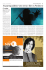### Hotrodden the Predator Part 2 of 3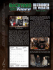### Hotrodden the Predator Part 1 of 3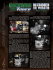### Internet Safety Guide for Teens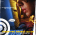### Do predators always kill substandard Individuals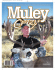### your wedding at predator ridge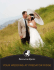### A minimal model of predator–swarm interactions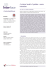### Lorenz system II - Harvard Mathematics Department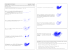### a FaNS GUIDE TO ScUlpTING, mOUlDING, caSTING aND paINTING a ScrEEN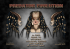### Predator Pain and Limitless: When Cybercrime Turns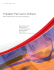### electronics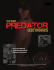### Mathematical Modeling of Disease Outbreak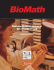### 2D Portraiture Unit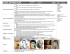### program overview - Art of Problem Solving Foundation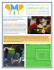### Pattern of money allocation in experimental games supports the